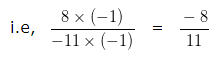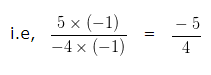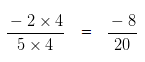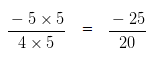# Comparing Rational Numbers

Comparing Rational Numbers – To compare any two Rational Number we have to follow the following steps:-

Step I: Express both the Rational numbers with positive Denominator.

Step II: Take the LCM of the positive Denominators.

Step III: Express both the Rational number obtained in step I with this LCM.

Step IV: The number with higher Numerator is greater.

### Comparing Rational Numbers Example 1

Fill the missing place:

12/13 ___ 10/13

Explanation

1st number = 12/13

2nd number = 10/13

If two rational numbers have common denominators, the number with higher Numerator is greater

If we compare their Numerators, we notice that

12 > 10

Accordingly 12/13 > 10/13

Hence, 12/13 > 10/13

### Comparing Rational Numbers Example 2

Fill the missing place:

(-3)/11 ___ 8/(-11)

Explanation

If we express both the Rational numbers with positive Denominator, we would get

1st number = (-3)/11

2nd number = 8/(-11)

To express 8/(-11) with a positive Denominator we would multiply both its Numerator and Denominator by (-1).If two rational numbers have common denominators, the number with higher Numerator is greater

If we compare their Numerators, we notice that

-3 > -8

then, (-3)/11 > (-8)/11

Hence, (-3)/11 > 8/(-11)

### Comparing Rational Numbers Example 3

Fill the missing place:

(-2)/5 ___ 5/(-4)

Explanation

If we express both the Rational numbers with positive Denominator, we would get

1st number = (-2)/5

2nd number = 5/(-4)

To express 5/(-4) with a positive Denominator we would multiply both its Numerator and Denominator by (-1).Since Denominators are different, we would need to solve the given equations in the following manner

Take LCM of 5 and 4 which is 20

Multiply both the Numerator and Denominator of (-2)/5 by 4 we would get .Similarly, Multiplying Numerator and Denominator of (-5)/4 by 5 .If two rational numbers have common denominators, the number with higher Numerator is greater

On comparing Numerators of (-8)/20 and (-25)/20

we find -8 > -25

then, (-8)/20 > (-25)/20

Hence, (-2)/5 > 5/(-4)